﻿ 不同优化算法在稳压器重量优化中的比较
«上一篇文章快速检索 高级检索

 应用科技2020, Vol. 47Issue (2): 98-103  DOI: 10.11991/yykj.2019050200

### 引用本文LI Chunmei, LI Yi, LIU Lizhi, et al. Comparison of different optimization algorithms in weight optimization of a pressurizer[J]. Applied Science and Technology, 2020, 47(2): 98-103. DOI: 10.11991/yykj.201905020.### 文章历史

Comparison of different optimization algorithms in weight optimization of a pressurizer
LI Chunmei, LI Yi, LIU Lizhi, HAO Chengming, SUN Guanyu, ZHANG Qi, WANG Yi, XIE Lidong, MIAO Yiran, XIA Junbao, YAN Siwei
Science and Technology on Reactor System Design Technology Laboratory, Nuclear Power Institute of China, Chengdu 610041, China
Abstract: In order to supply a feasible method to optimize the design of nuclear power plant, the case study on the weight optimization of pressurizer, which is one of the key devices in nuclear power system with pressurized water reactor, is performed in this paper. The improved complex algorithm, genetic algorithm and particle swam algorithm are incorporated in the optimization case study as representative algorithms. Such performance as global optimization, convergence, stability and efficiency are compared among three selected algorithms. The results show that in aspect of the pressurizer weight optimization, the genetic algorithm can find global optimization scheme in greater possibility as well as the particle swam algorithm, while the improved complex algorithm takes the advantage in aspects of convergence and efficiency. The findings in this paper are beneficial to the optimization of nuclear power plant.
Keywords: pressurizer    design optimization    weight optimization    improved complex algorithm    genetic algorithm    particle swam algorithm    optimization of nuclear power plant    convergence

1 稳压器计算模型

1.1 稳压器容积计算模型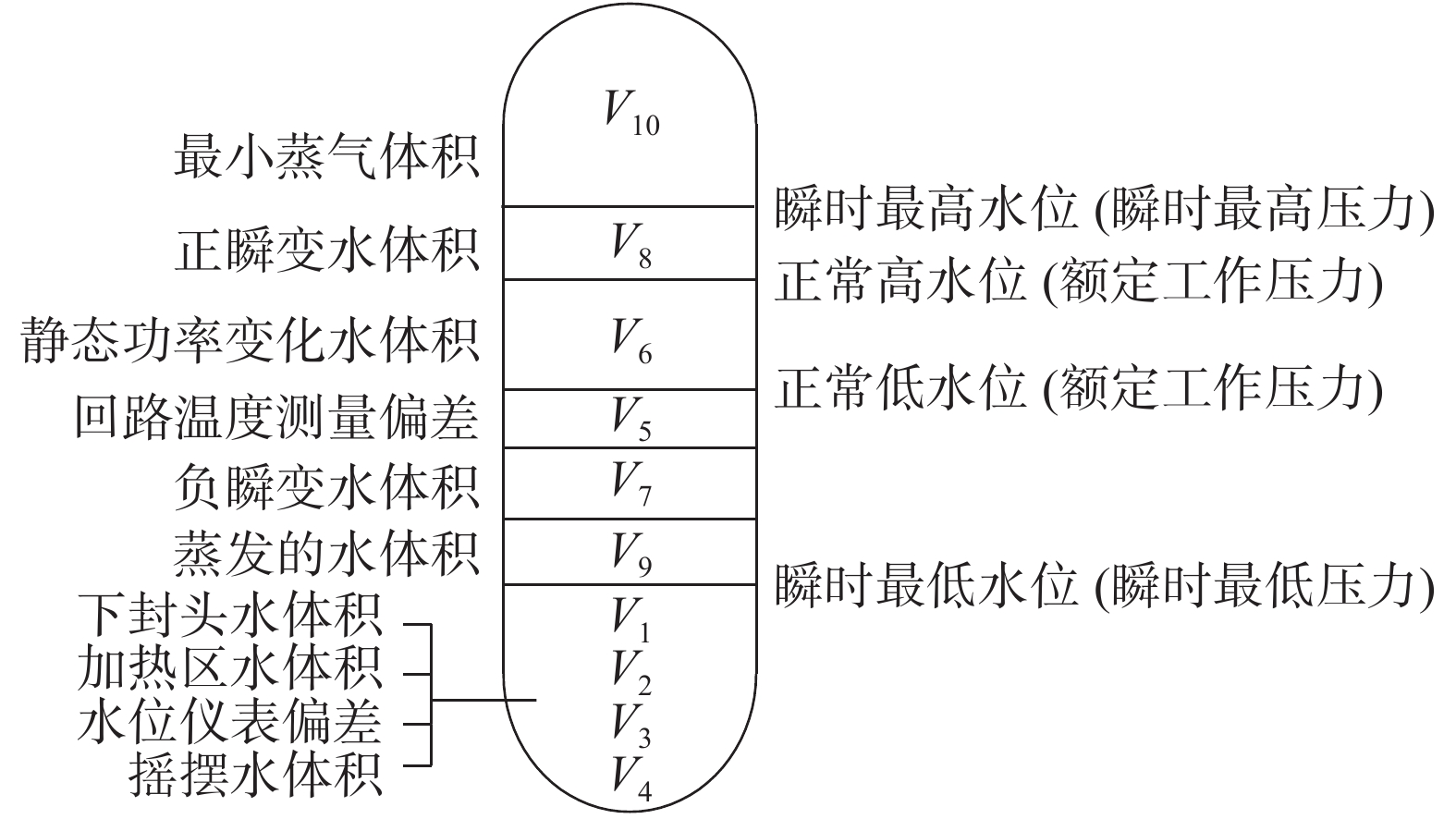Download: 图 1 稳压器内部容积构成示意
1.2 稳压器重量计算模型

1.2.1 封头壁厚计算

 ${\delta _1} = \frac{{PDk}}{{2[\sigma ]\varphi - 0.5P}}$

 ${\delta _2} = \frac{{PD}}{{2[\delta ]\varphi - P}}$ (1)

 ${\delta _3} = \frac{{PD}}{{2[\delta ]r - P}}$

1.2.2 稳压器重量的计算

1）若稳压器采用椭圆封头，则其重量可由式（2）确定：

 ${M_1} = \left\{ {\frac{{{\rm{2{\text{π}} }}{{(D + 2{\delta _1})}^2}}}{{\rm{3}}}{{h'}_1} - \frac{{{\rm{2{\text{π}} }}{D^2}}}{{\rm{3}}}{h_{\rm{1}}}} \right\}\rho$ (2)

 ${M_1} = \left\{ {\frac{{{\text{π}}}}{6}\left[ {{{\left( {D + 2{\delta _1}} \right)}^3} - {D^3}} \right]} \right\}\rho$

 ${M_1} = \left\{ {\frac{{2{{\text{π}}}}}{3}\left[ {{{\left( {D + 2{\delta _1}} \right)}^3} - {D^3}} \right]} \right\}\rho$

2）圆柱筒体重量

 $\begin{array}{l} {M_2} = \dfrac{{{\text{π}}}}{4}\left[ {{{\left( {D + 2{\delta _2}} \right)}^2} - {D^2}} \right]{H_y}\rho \\ \;\;\;\;{H_y} = {H_{{\rm{PR}}}} - 2{H_s} - {H_2} \end{array}$

3）电加热区筒体重量

 ${M_3} = \frac{{{\text{π}}}}{4}\left[ {{{\left( {D + 2{\delta _3}} \right)}^2} - {D^2}} \right]{H_2}\rho$

4）稳压器总重量

 $M = 2{M_1} + {M_2} + {M_3}$

 ${M_{{\rm{PR}}}} = 2{M_1} + {M_2} + {M_3} + {M_4}$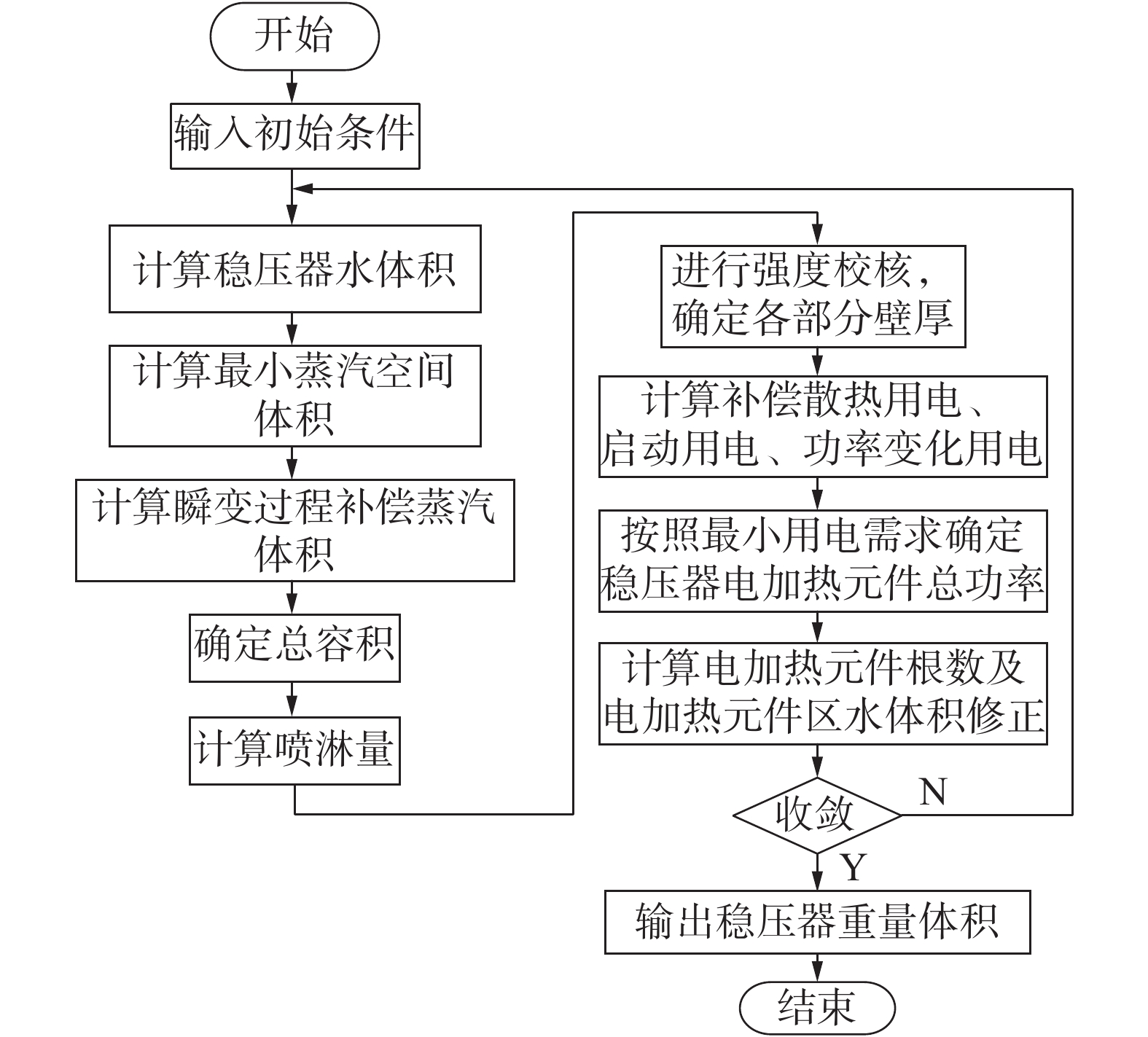Download: 图 2 稳压器设计计算流程
2 优化算法 2.1 复合形算法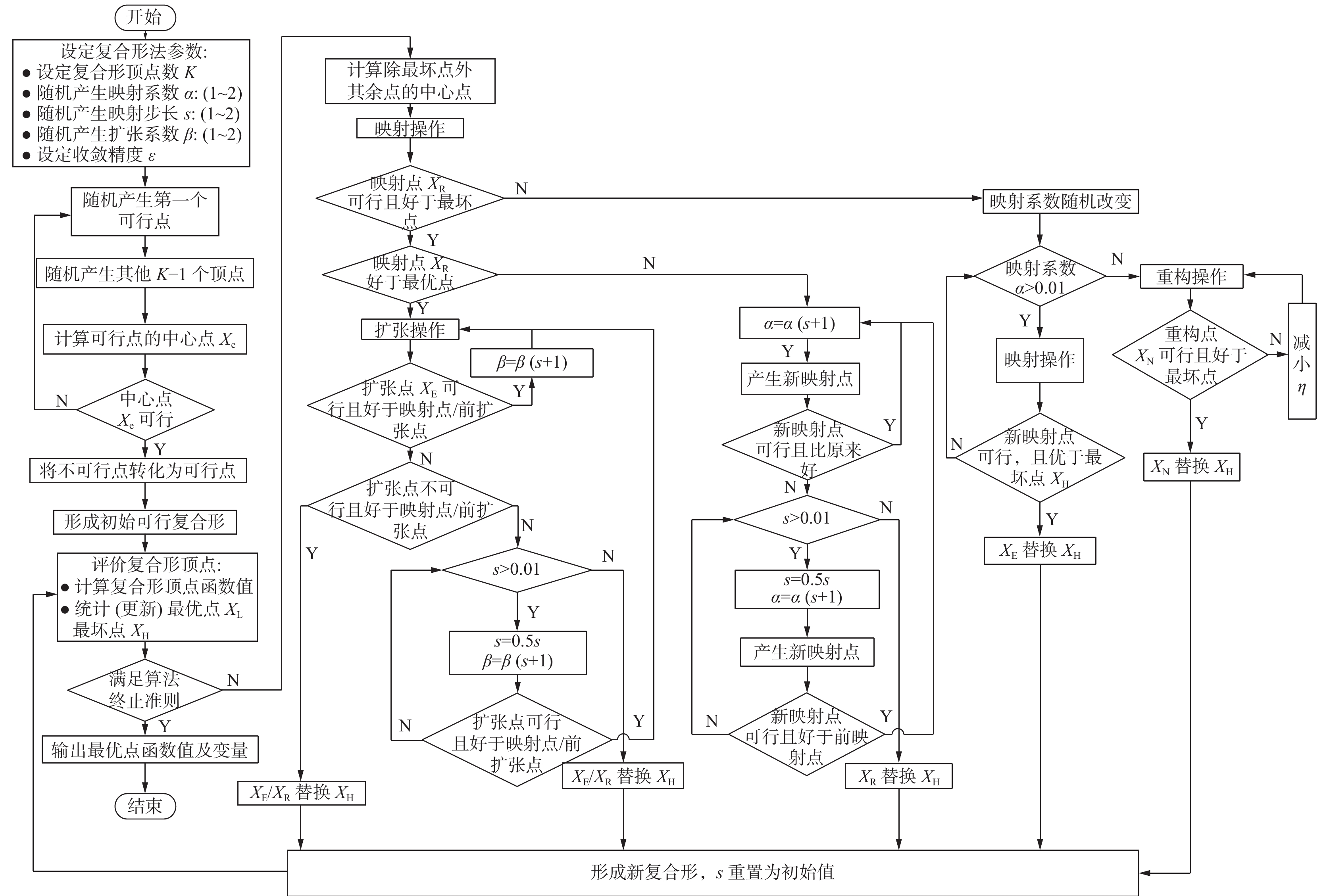Download: 图 3 改进复合形算法流程
2.2 遗传算法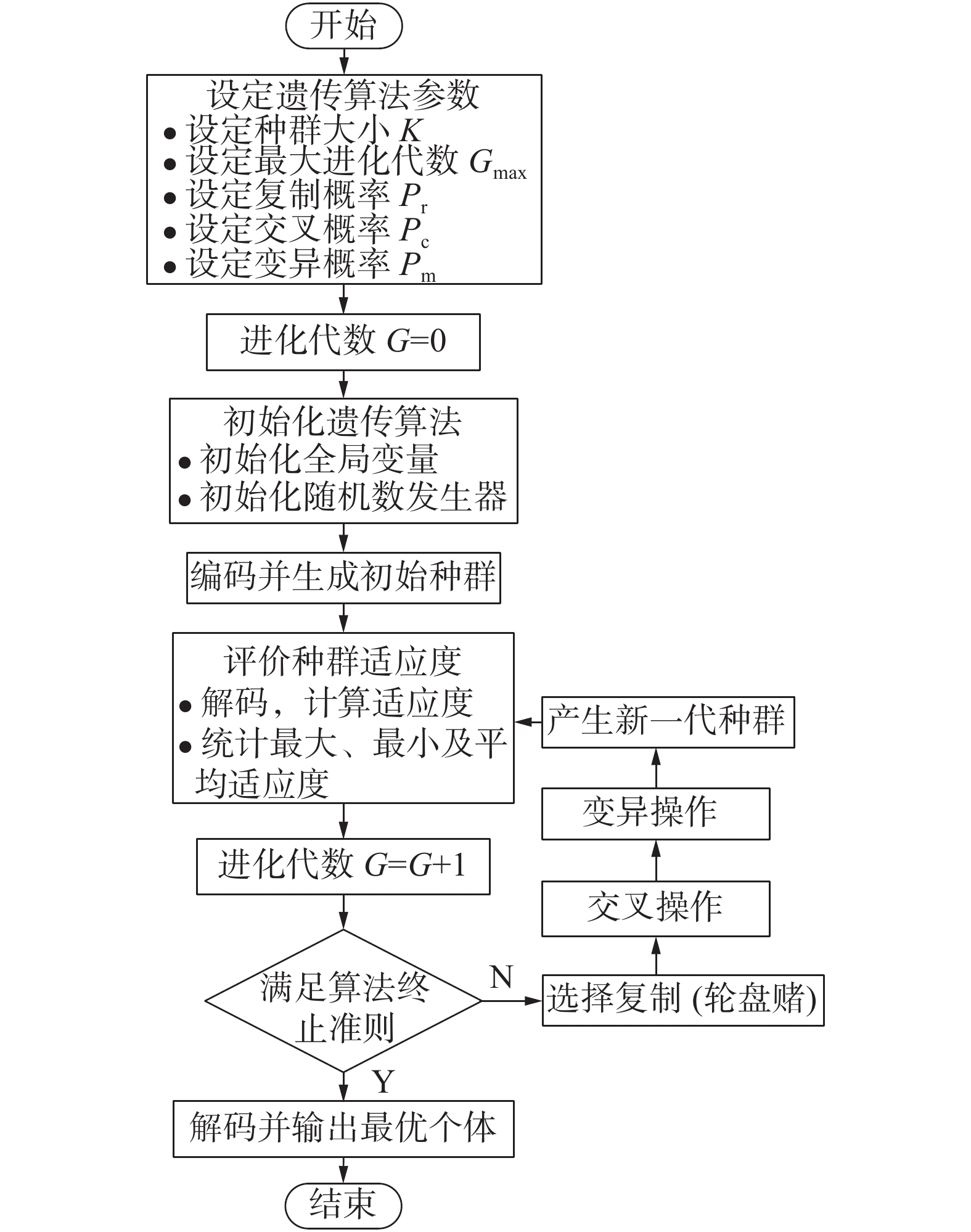Download: 图 4 遗传算法计算流程
2.3 粒子群算法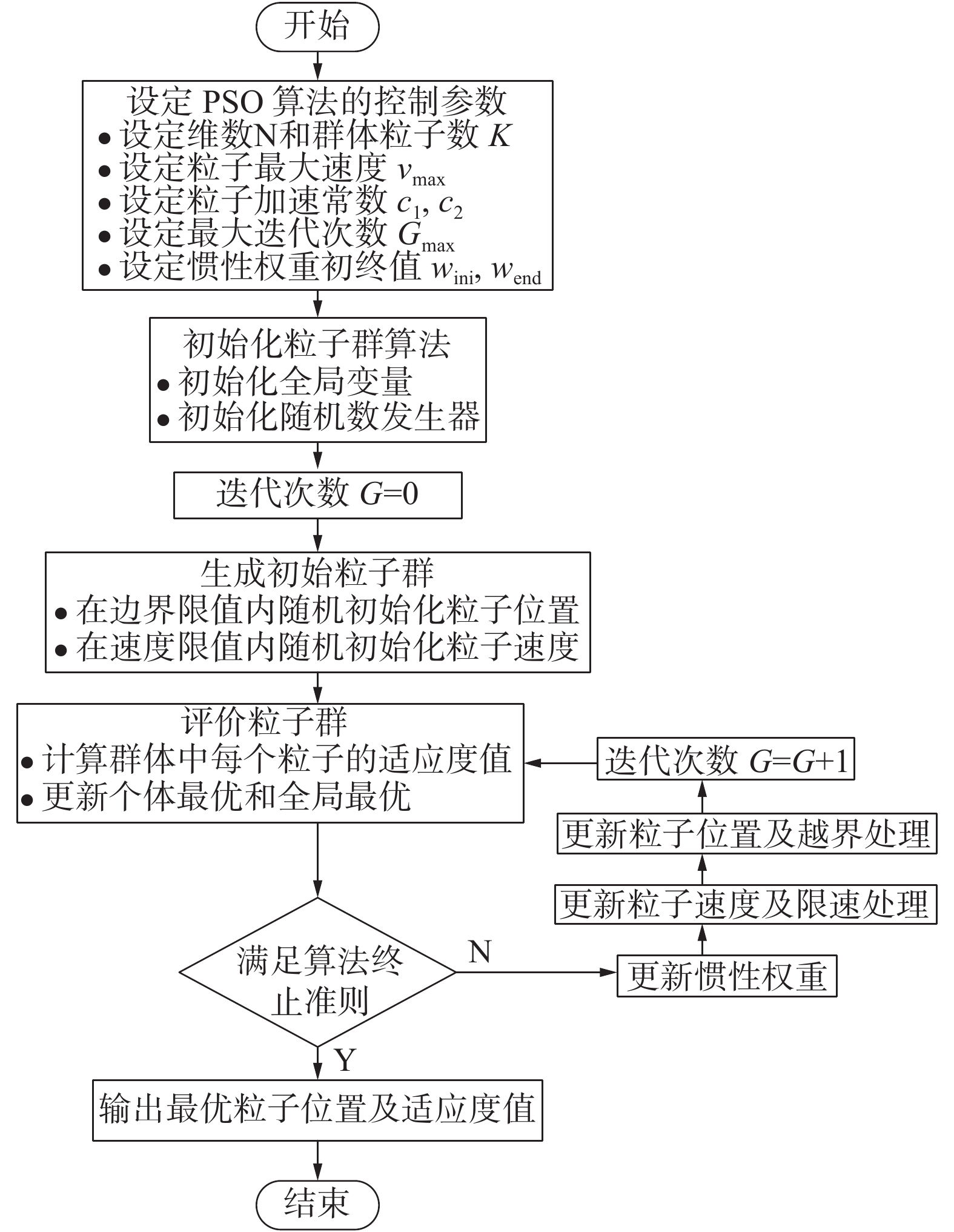Download: 图 5 粒子群算法流程
3 稳压器重量优化问题

 ${{M}}({{{X}}_{{\rm{opt}}}}) = \min [M({{X}})],\forall {{X}} \in { R}$ (3)

4 结果与讨论 4.1 敏感性分析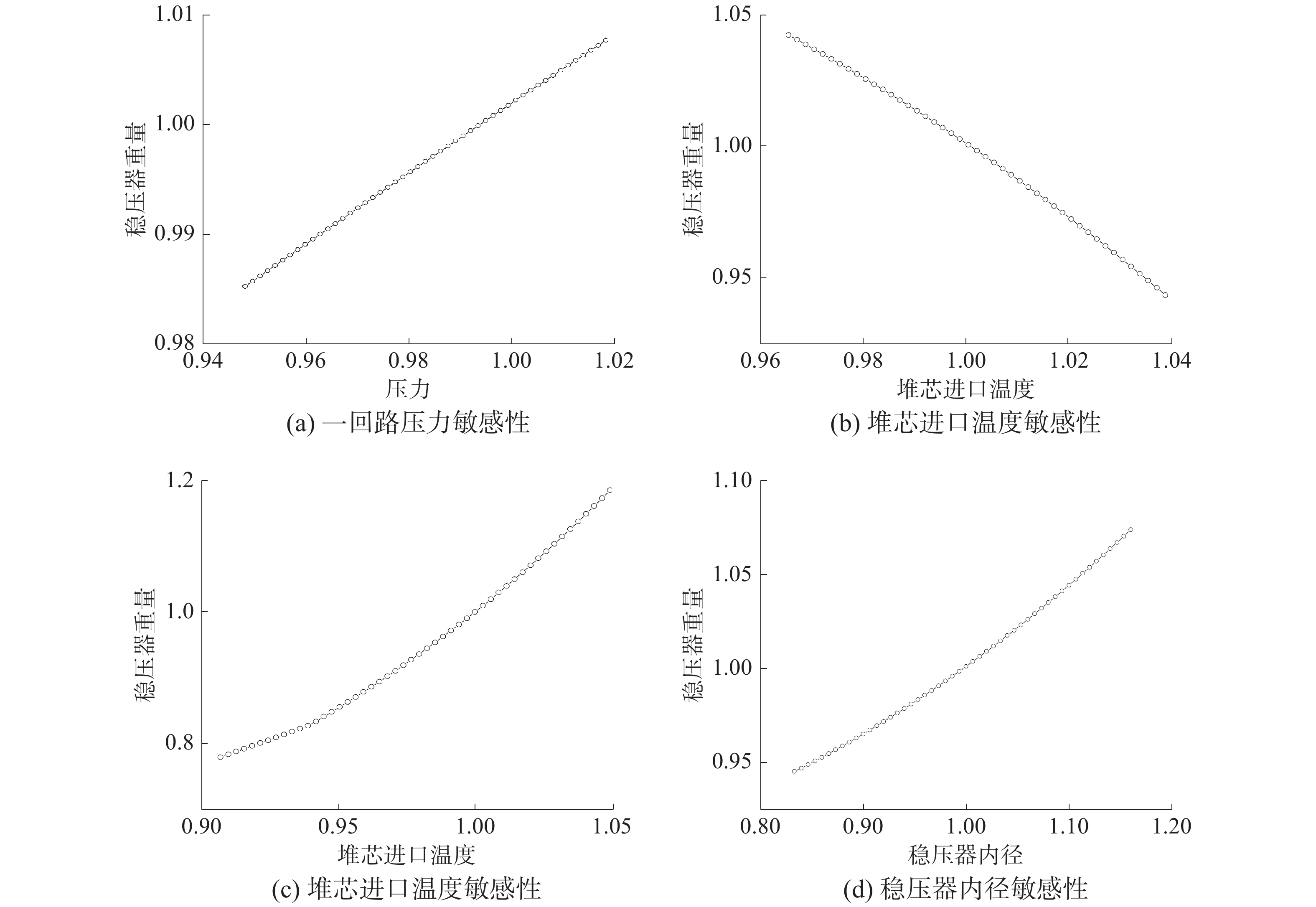Download: 图 6 敏感性分析结果（图中数据均经过归一化处理）

4.2 优化计算结果比较表 1 3种算法计算结果比较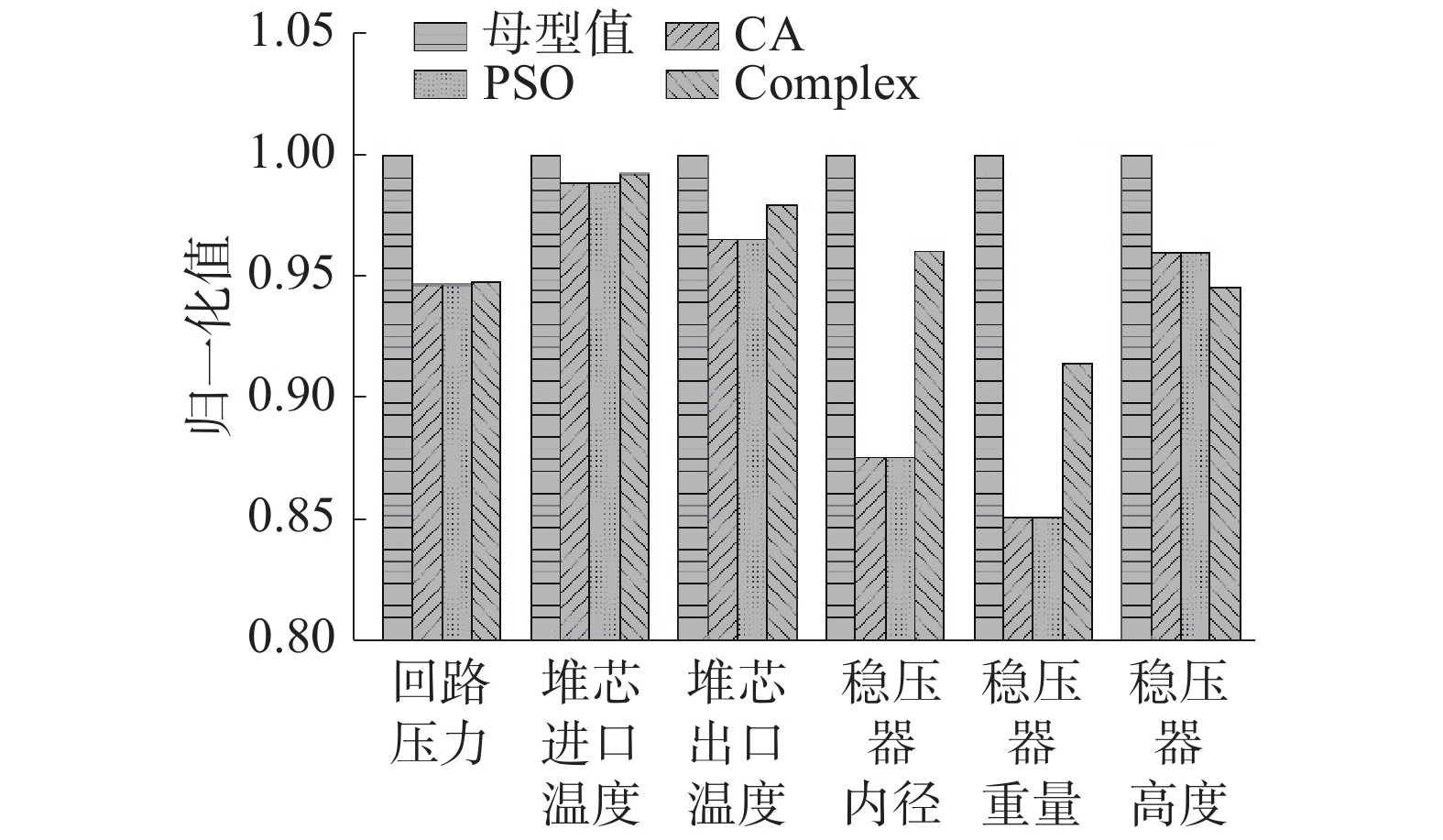Download: 图 7 不同算法优化结果与母型参数对比
5 结论

1）对于稳压器重量最小目标的优化设计，改进复合形算法的计算效率最高，但存在初值依赖问题；

2）对于稳压器重量最小目标的优化设计，粒子群算法与遗传算法表现出良好的一致性，这2种算法均能在全局范围内找到优化解；

3）综合来看，粒子群算法是最适用于稳压器重量最小目标的优化设计方法，可在单一核动力设备优化设计中推广应用。

  贺士晶, 阎昌琪, 王建军, 等. 稳压器容积的优化设计[J]. 原子能科学技术, 2010, 44(3): 320-324. (0)  孙中宁. 核动力设备[M]. 哈尔滨: 哈尔滨工程大学出版社, 2003: 101-135. (0)  贺士晶. 核动力装置参数优化设计[D]. 哈尔滨: 哈尔滨工程大学, 2010. (0)  马秉骞. 椭圆形封头和碟形封头强度计算公式的应用条件[J]. 石油和化工设备, 2008, 11(1): 27-28. DOI:10.3969/j.issn.1674-8980.2008.01.007 (0)  朱齐荣. 核电厂机械设备及其设计[M]. 北京: 原子能出版社, 2000: 53-110. (0)  孙靖民, 梁迎春. 机械优化设计[M]. 北京: 机械工业出版社, 2006: 130-135. (0)  王盟, 王建军, 贺士晶, 等. 一种解决复合形局部最优及加速计算的方法[J]. 计算机应用与软件, 2011, 28(2): 277-278, 289. DOI:10.3969/j.issn.1000-386X.2011.02.083 (0)  刘成洋, 阎昌琪, 王建军. 基于改进遗传算法的冷凝器优化设计[J]. 原子能科学技术, 2012, 46(4): 457-462. (0)  李贵敬, 阎昌琪, 王建军, 等. 映射交叉遗传算法在核动力设备优化设计中的应用[J]. 原子能科学技术, 2013, 47(7): 1212-1216. DOI:10.7538/yzk.2013.47.07.1212 (0)  LAPA C M F, PEREIRA C M N A, DE A. MOL A C. Maximization of a nuclear system availability through maintenance scheduling optimization using a genetic algorithm[J]. Nuclear engineering and design, 2000, 196(2): 219-231. DOI:10.1016/S0029-5493(99)00295-2 (0)  周明, 孙树栋. 遗传算法原理及应用[M]. 北京: 国防工业出版社, 1999: 4-7. (0)  刘波. 粒子群优化算法及其工程应用[M]. 北京: 电子工业出版社, 2010: 1–2. (0)  PEREIRA C M N A, LAPA C M F, MOL A C A, et al. A particle swarm optimization (PSO) approach for non-periodic preventive maintenance scheduling programming[J]. Progress in nuclear energy, 2010, 52(8): 710-714. DOI:10.1016/j.pnucene.2010.04.009 (0)